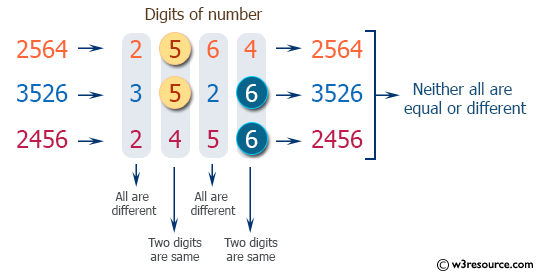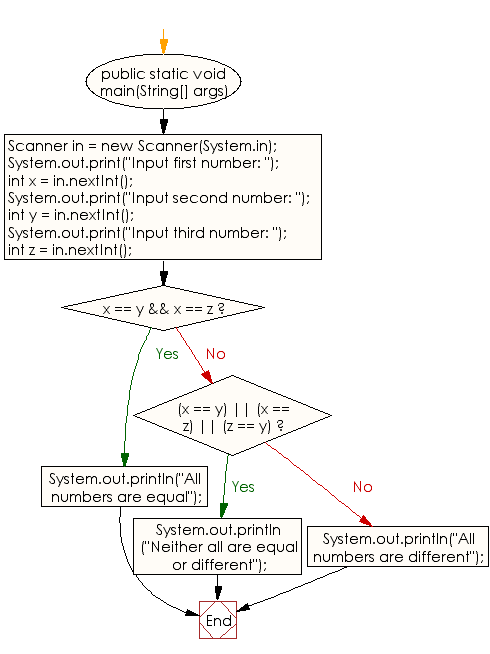﻿ Java exercises: Accepts three numbers and prints - w3resource# Java Conditional Statement Exercises: Accepts three numbers and prints

## Java Conditional Statement: Exercise-30 with Solution

Write a Java program that accepts three numbers and prints "All numbers are equal" if all three numbers are equal, "All numbers are different" if all three numbers are different and "Neither all are equal or different" otherwise.

Test Data
Input first number: 2564
Input second number: 3526
Input third number: 2456

Pictorial Presentation:Sample Solution:

Java Code:

``````import java.util.Scanner;
public class Exercise30 {

public static void main(String[] args)
{
Scanner in = new Scanner(System.in);

System.out.print("Input first number: ");
int x = in.nextInt();
System.out.print("Input second number: ");
int y = in.nextInt();
System.out.print("Input third number: ");
int z = in.nextInt();

if (x == y && x == z)
{
System.out.println("All numbers are equal");
}
else if ((x == y) || (x == z) || (z == y))
{
System.out.println("Neither all are equal or different");
}
else
{
System.out.println("All numbers are different");
}
}
}
```
```

Sample Output:

```Input first number: 2564
Input second number: 3526
Input third number: 2456
All numbers are different
```

Flowchart:Java Code Editor: